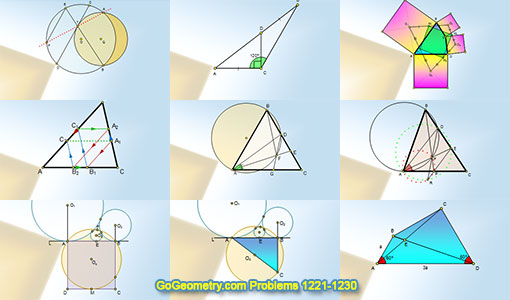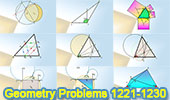# Geometry Problems 1221-1230. Level: High School, CollegeGeometry Problems 1221 - 1230Geometry Problem 1221 Intersecting Circles, Chord, Tangent, Parallel Chords, Collinear Points. Geometry Problem 1222 Doubling the Cube, Triangle, 90, 120 degrees, Perpendicular, Congruence. Geometry Problem 1223 Circle, Parallel Chords. Geometry Problem 1224 Triangle, Parallel to the Sides. Geometry Problem 1225 Equilateral Triangle, Circumcircle, Angle Bisector, Congruence. Geometry Problem 1226 Quadrilateral, Squares, Centers, Midpoints, Congruence. Geometry Problem 1227 Triangle, Circumcircle, Angle Bisector, Concyclic Points, Cyclic Quadrilateral. Geometry Problem 1228 Three Tangent Circles, Midpoint, Major Arc, Common Tangent Line, Square. Geometry Problem 1229 Three Tangent Circles, Common Tangent Line, 3-4-5 Right Triangle. Geometry Problem 1230 Quadrilateral, 60 Degrees, Congruence, Metric Relations.

Home | Sitemap | Search | Geometry | Problems | Visual Index | 10 ProblemsEmail | Post a comment | by Antonio Gutierrez
Last updated: Feb 1, 2017